2. Templates - Fields

# Number

## Field to enter numerical values.

If we want to insert numerical quantities in the form, we can use the Number type field. In addition to numbers, the user can write characters such as "+" or "-" and the number "e", among others.

When moving the mouse over the field, two arrows will appear that will allow us to increase or decrease the numerical value.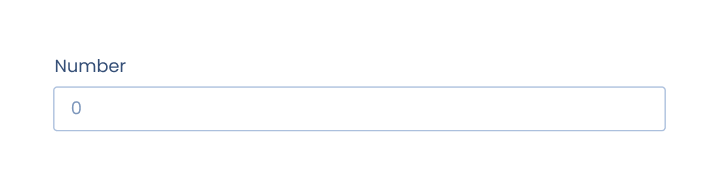### Description of the Number field options

• Title: phrase or question that describes the purpose of the field.
• Tag: we must introduce the same tag that we have written in the "template document" so that the field is correctly linked with its position in the document.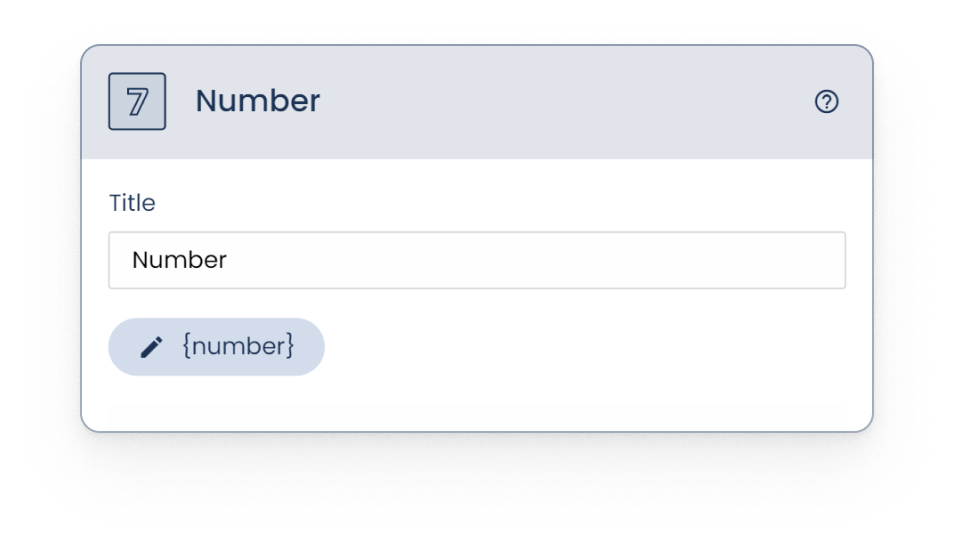1. Subtitle: used in case we want to add additional information to the title.

2. Optional field: used to make the field required or optional. When activated, we will be telling the software that this field, despite being asked, will not necessarily have to be answered.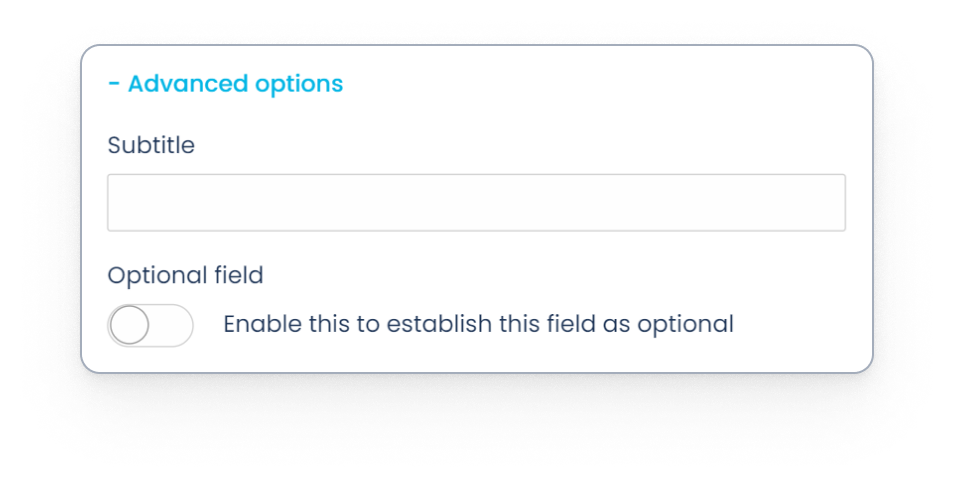3. Blocked field: Enabling this option will restrict the field to be automatically populated with predefined data, whether by internal means (copying data from another document) or external (bulk document creation, integrations, API...).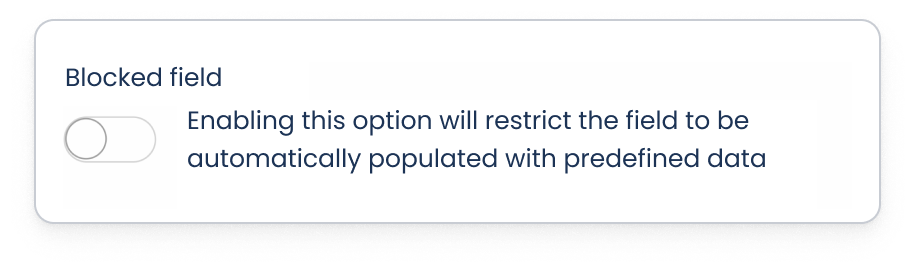4. Placeholder: allows you to enter a provisional text that will be displayed inside the field in the form -simulating a response- and will disappear when filling it with the final data.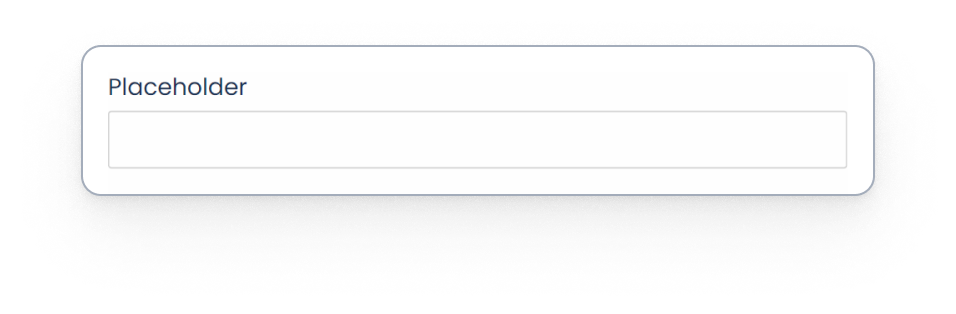5. Select type: this choice will not impact how the question will be displayed in the form, but rather how the answers will appear in the final document.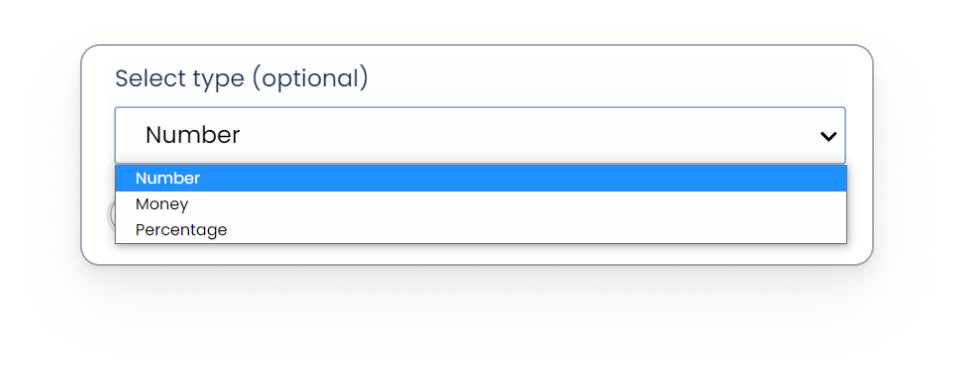The different types of numeric field are as follows:

• Number: for numerical quantities, in general. Depending on the language of the template, decimals and thousands will be indicated in one way or another. For example, in English, decimals will be separated by a period (.) and thousands by a comma (,).

If we want it to appear in one or more languages ​​other than the one in the template, we must indicate it in the field tag itself, when writing it in the template, adding an underscore (_) and the abbreviation of the corresponding language:

English: {field_EN}
Spanish: {field_ES}
German: {field_DE}
Austrian German: {field_DE_AT}
Catalan: {field_CA}
French: {field_FR}
Italian: {field_IT}
Portuguese: {field_PT}

Then, for the platform to correctly identify the tags, we must select all the languages used through the "Multilanguage" dropdown, in the template settings section: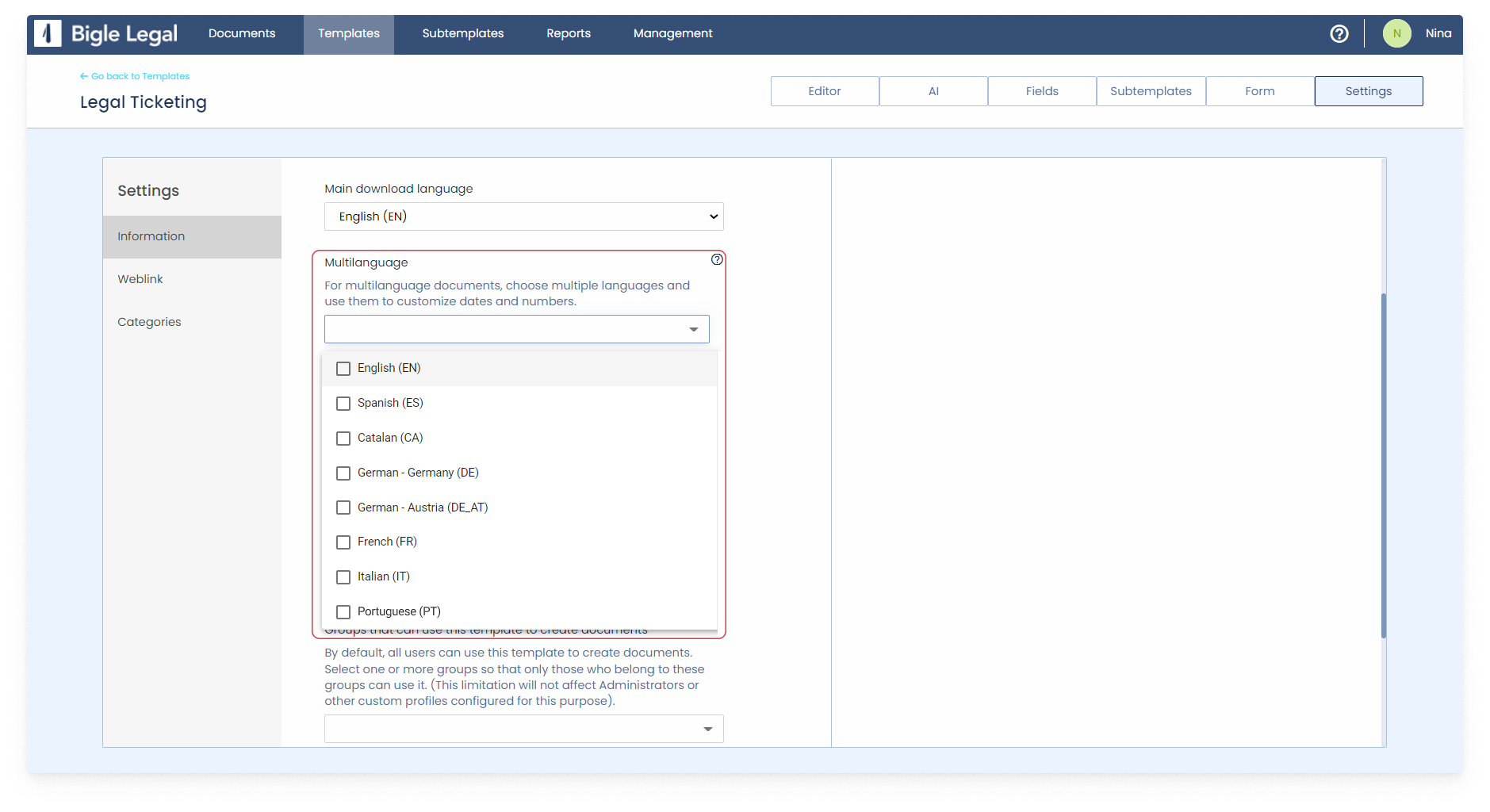• Percentage: the percentage is expressed using the % symbol. It is written after the number to which it refers.
• Money: for cases in which the amount is monetary. It transforms the form of numeric representation into the chosen currency and format.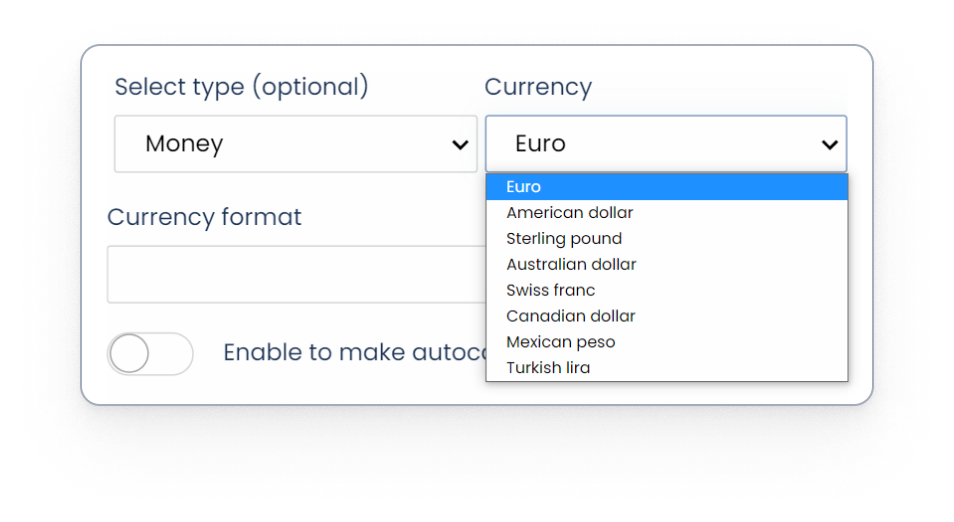6. Autocalculation: allows us to make the numerical value of the field be calculated automatically.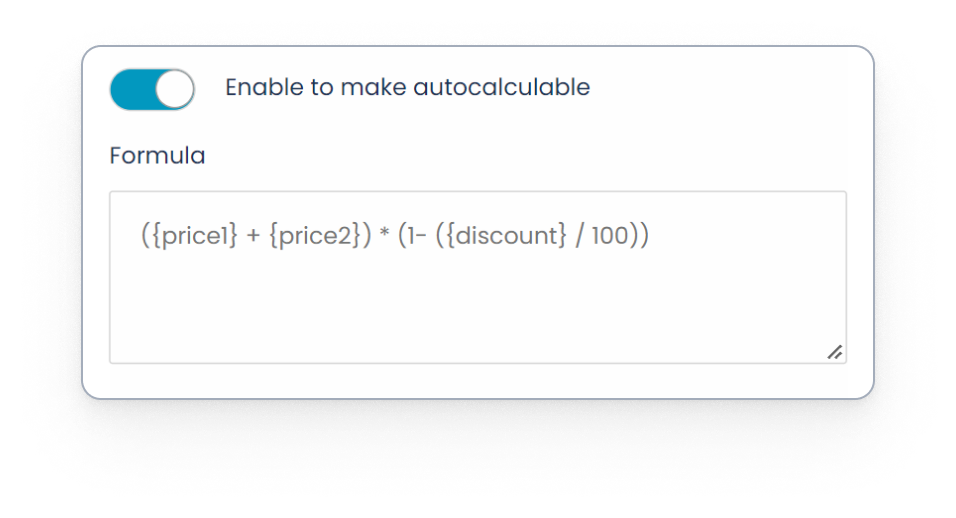For this to happen, we must enter a valid formula in the "Formula" field that we will also find within the advanced options.

The formula can contain tags that refer to other fields already created, even if they also use autocalculation (as long as they do not use each other to calculate their values). The available valid fields will be suggested by the platform when creating the formula.

For example, the value of a certain final price could be calculated automatically, as a result of applying a discount to the sum of two different prices, with a formula like this:

({price1} + {price2}) * (1- ({discount} / 100))

In the event that the entered formula does not work correctly or generates a different value than expected, we will have to check the following:

That the formula is valid and well written.
That the fields used in the formula exist.
That in the formula we do not use any field that is in a multiple section, or in a subsection within a multiple section.
That said formula does not conflict with other formulas in the field or in the same template, creating a circular reference. This can happen if, for example, in a template we have three fields with autocalculation whose formulas refer to each other:

- Autocalculus A ({autocalculusA}): {fieldA} + {autocalculusB}
- Autocalculus B ({autocalculusB}): {fieldB} + {autocalculusC}
- Autocalculus C ({autocalculusC}): {fieldC} + {autocalculusA}

Analyzing the previous case, we see that “{autocalculusA} = {fieldA} + {fieldB} + {fieldC} + {autocalculusA}”. To calculate the value of “{autocalculusA}” we end up using “{autocalculusA}” itself, so this formula, or any other one in which a case like this occurs, would not be valid.

7. Minimum and maximum: they allow the numerical answer to be compressed between two numbers. If the entered number does not meet this requirement, an error message will appear.

8. Enable decimals: this feature allows the platform to display decimals.

9. Number of decimals: to set the maximum number of decimals allowed (if we have previously activated decimals).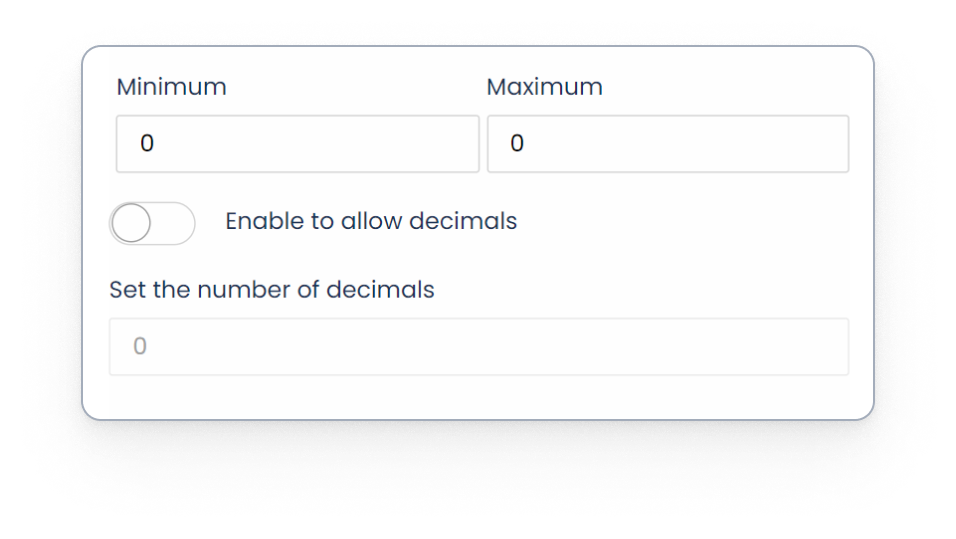10. Download options: we can make the numbers generated or entered in the form appear written in letters in the final document. To do this, we must use number-to-word transformation tags (learn how to configure them in this article). If we opt for this possibility, in the download options we can specify if we want the words that describe the numerical values to appear capitalized, in lowercase or in uppercase.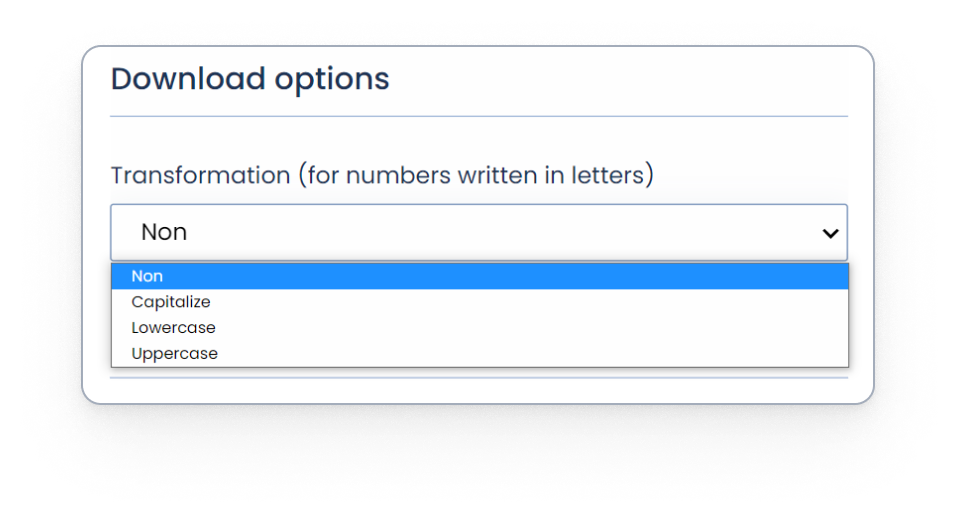11. Explanation: if we activate this option, we can add detailed instructions or explanations that serve as support when filling in the field in the form.

12. Form metadata: if enabled, the values entered in this field will be saved and used as metadata.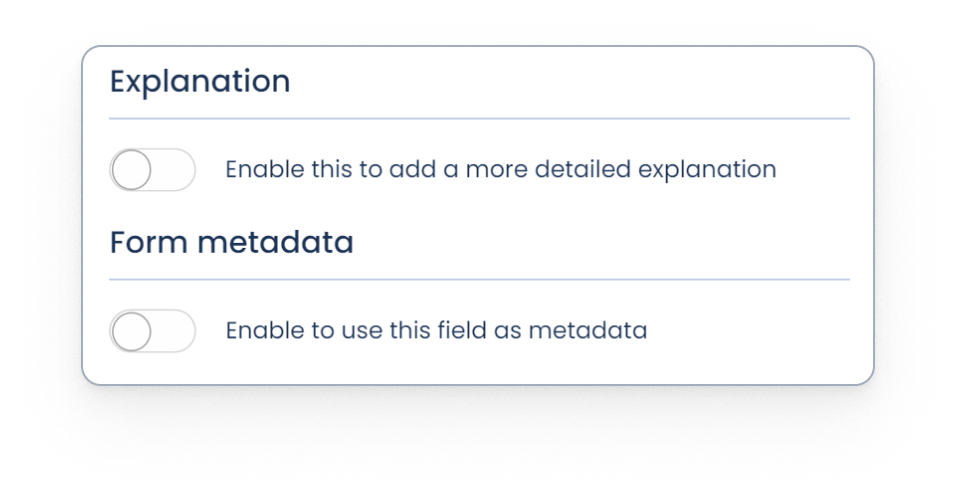As an additional functionality, the platform offers us the possibility of configuring a Number type field so that, depending on the value entered in the form, one sentence or another is displayed in the final document.

Learn how to configure tags to condition texts to the value of a numeric field in this article.

Once the field is set up, save the changes. The card folds, showing us information with which to check the basic configuration of the field in the list of fields: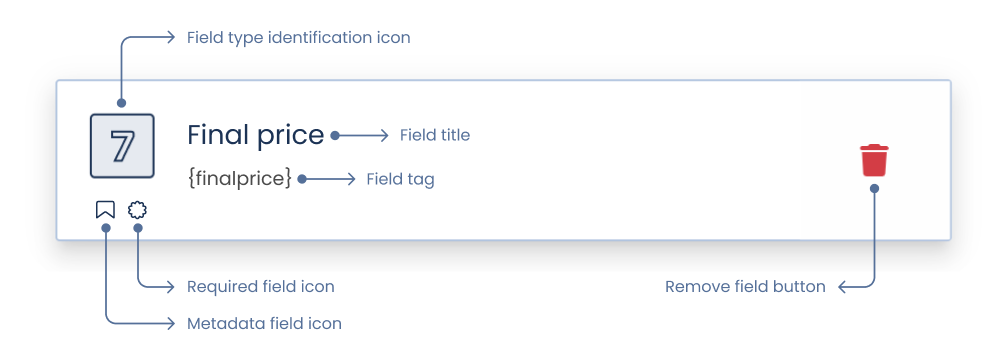Related articles:

Short text

Long text

Date

Single choice

Multiple choice

Dropdown